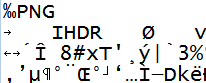# Packing & Unpacking: A Guide to Reading Binary Data in Ruby

But why do we need these methods?

Working with text is a lot easier than working with binary data.

Text allows you to use:

But if you want to work with binary data, there is some extra work to do. That’s where the Array#pack & String#unpack methods come into play.

Let me show you some examples, starting with just a plain string & then moving on to more interesting stuff.

## String to ASCII Values

This will convert every character in the string into a decimal value:

```str = "AABBCC"
str.unpack("c*")

# [65, 65, 66, 66, 67, 67]
```

Notice the `"c*"` argument for `unpack`.

This is a “format string” which tells `unpack` what to do with the data. In this case, `c` means take one character & convert it into an integer value (the String#ord method also does this).

The asterisk `*` just says “repeat this format for all the input data”.

## Convert Hex to String

The `H` used with the `pack` method gives you a hex number to string conversion.

Example:

```["414243"].pack("H*")
# "ABC"

"ABC".unpack("H*")
# ["414243"]
```

## How to Convert Hex to Integer

This format string takes 4 bytes of data & returns an integer. One thing to notice is that these bytes are in “little-endian” format.

Examples:

```"\xff\x00\x00\x00".unpack("l").first
# 255
```
```"\x90\xC0\xDD\x08".unpack("l").first
# 148750480
```

I used `first` here because `unpack` returns an array.

## Binary File Parsing With The Unpack Method

How do you read a binary file like an EXE, PNG or GZIP?

If you read these files like plain text, you’ll see something that looks like random data…It’s not random stuff.

There is a documented structure for many of these file formats & the `unpack` method is what you can use to read that data and convert it into something useful.

Here is an example:

```binary_data     = "\x05\x00\x68\x65\x6c\x6c\x6f"
length, message = binary_data.unpack("Sa*")

# [5, "hello"]
```

In this example, the binary data (represented in hexadecimal, which is way more compact than 1s & 0s) has a two-byte (16 bit) length field that contains the length of the following string. Then there is the string itself.

It is very common for binary files & binary network protocols to have a “length” field.

This tells the parser exactly how many bytes should be read.

Yes.

I know in this example I read both the length & the data in one step, that’s just to keep things simple.

## How to Use The BinData Gem

There is also the bindata gem, which is built specifically to help you parse binary structures.

Here is an example:

```class BinaryString < BinData::Record
endian :little
uint16 :len
end
```

Notice the `read_length` parameter. This will tell bindata to work out the length from the field, so this will save you a lot of work 🙂

So if you want to write a parser for any binary format, these are the steps:

1. Find the specification for this format (if it’s not public you will have to reverse-engineer it, which is an entire topic on its own)
2. Write a `bindata` class for every section of the file (you will usually find a header section first with metadata & then multiple data sections)
3. Read the data & process it however you want (for example, in a PNG you could change the colors of the image)
4. Profit!

If you want to see a full example of `bindata` in action take a look at my PNG parser on github.

## Base64 Encoding

There is this type of encoding called “Base64”. You may have seen it before on a URL.

Looks something like this:

```U2VuZCByZWluZm9yY2VtZW50cw==
```

The double equals at the end is usually the tell-tale sign that you are dealing with `Base64`, although some inputs can result in the equals signs not being there (they are used as padding).

So why I’m telling you this…

Besides being a useful thing to know by itself?

Well, it turns out that you can convert a string into `Base64` using the `pack` method.

As you can see here:

```def encode64(bin)
[bin].pack("m")
end

encode64 "abcd"

# "YWJjZA==\n"
```

In fact, this is the exact method used in the `Base64` module from the standard library.

## Summary

In this post, you learned about the `pack` & `unpack` methods, which help you work with binary data. You can use this to parse binary files, convert a string into ASCII values, and for Base64 encoding.

Don’t forget to share & subscribe so you can enjoy more blog post like this! 🙂